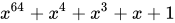# Cyclic redundancy check

A cyclic redundancy check (CRC) is an error-detecting code commonly used in digital networks and storage devices to detect accidental changes to digital data. Blocks of data entering these systems get a short check value attached, based on the remainder of a polynomial division of their contents. On retrieval, the calculation is repeated and, in the event the check values do not match, corrective action can be taken against data corruption. CRCs can be used for error correction (see bitfilters).

CRCs are so called because the check (data verification) value is a redundancy (it expands the message without adding information) and the algorithm is based on cyclic codes. CRCs are popular because they are simple to implement in binary hardware, easy to analyze mathematically, and particularly good at detecting common errors caused by noise in transmission channels. Because the check value has a fixed length, the function that generates it is occasionally used as a hash function.

## Introduction

CRCs are based on the theory of cyclic error-correcting codes. The use of systematic cyclic codes, which encode messages by adding a fixed-length check value, for the purpose of error detection in communication networks, was first proposed by W. Wesley Peterson in 1961. Cyclic codes are not only simple to implement but have the benefit of being particularly well suited for the detection of burst errors: contiguous sequences of erroneous data symbols in messages. This is important because burst errors are common transmission errors in many communication channels, including magnetic and optical storage devices. Typically an n-bit CRC applied to a data block of arbitrary length will detect any single error burst not longer than n bits, and the fraction of all longer error bursts that it will detect is (1 − 2n).

Specification of a CRC code requires definition of a so-called generator polynomial. This polynomial becomes the divisor in a polynomial long division, which takes the message as the dividend and in which the quotient is discarded and the remainder becomes the result. The important caveat is that the polynomial coefficients are calculated according to the arithmetic of a finite field, so the addition operation can always be performed bitwise-parallel (there is no carry between digits).

In practice, all commonly used CRCs employ the Galois field, or more simply a finite field, of two elements, GF(2). The two elements are usually called 0 and 1, comfortably matching computer architecture.

A CRC is called an n-bit CRC when its check value is n bits long. For a given n, multiple CRCs are possible, each with a different polynomial. Such a polynomial has highest degree n, which means it has n + 1 terms. In other words, the polynomial has a length of n + 1; its encoding requires n + 1 bits. Note that most polynomial specifications either drop the MSB or LSB, since they are always 1. The CRC and associated polynomial typically have a name of the form CRC-n-XXX as in the table below.

The simplest error-detection system, the parity bit, is in fact a 1-bit CRC: it uses the generator polynomial x + 1 (two terms), and has the name CRC-1.

## Application

A CRC-enabled device calculates a short, fixed-length binary sequence, known as the check value or CRC, for each block of data to be sent or stored and appends it to the data, forming a codeword.

When a codeword is received or read, the device either compares its check value with one freshly calculated from the data block, or equivalently, performs a CRC on the whole codeword and compares the resulting check value with an expected residue constant.

If the CRC values do not match, then the block contains a data error.

The device may take corrective action, such as rereading the block or requesting that it be sent again. Otherwise, the data is assumed to be error-free (though, with some small probability, it may contain undetected errors; this is inherent in the nature of error-checking).

## Data integrity

CRCs are specifically designed to protect against common types of errors on communication channels, where they can provide quick and reasonable assurance of the integrity of messages delivered. However, they are not suitable for protecting against intentional alteration of data.

Firstly, as there is no authentication, an attacker can edit a message and recompute the CRC without the substitution being detected. When stored alongside the data, CRCs and cryptographic hash functions by themselves do not protect against intentional modification of data. Any application that requires protection against such attacks must use cryptographic authentication mechanisms, such as message authentication codes or digital signatures (which are commonly based on cryptographic hash functions).

Secondly, unlike cryptographic hash functions, CRC is an easily reversible function, which makes it unsuitable for use in digital signatures.

Thirdly, CRC satisfies a relation similar to that of a linear function (or more accurately, an affine function):

$\operatorname {CRC} (x\oplus y)=\operatorname {CRC} (x)\oplus \operatorname {CRC} (y)\oplus c$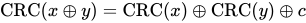where $c$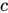depends on the length of $x$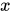and $y$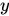. This can be also stated as follows, where $x$, $y$and $z$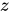have the same length

$\operatorname {CRC} (x\oplus y\oplus z)=\operatorname {CRC} (x)\oplus \operatorname {CRC} (y)\oplus \operatorname {CRC} (z);$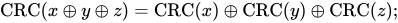as a result, even if the CRC is encrypted with a stream cipher that uses XOR as its combining operation (or mode of block cipher which effectively turns it into a stream cipher, such as OFB or CFB), both the message and the associated CRC can be manipulated without knowledge of the encryption key; this was one of the well-known design flaws of the Wired Equivalent Privacy (WEP) protocol.

## Computation

To compute an n-bit binary CRC, line the bits representing the input in a row, and position the (n + 1)-bit pattern representing the CRC's divisor (called a "polynomial") underneath the left end of the row.

In this example, we shall encode 14 bits of message with a 3-bit CRC, with a polynomial x3 + x + 1. The polynomial is written in binary as the coefficients; a 3rd-degree polynomial has 4 coefficients (1x3 + 0x2 + 1x + 1). In this case, the coefficients are 1, 0, 1 and 1. The result of the calculation is 3 bits long, which is why it is called a 3-bit CRC. However, you need 4 bits to explicitly state the polynomial.

11010011101100


This is first padded with zeros corresponding to the bit length n of the CRC. This is done so that the resulting code word is in systematic form. Here is the first calculation for computing a 3-bit CRC:

11010011101100 000 <--- input right padded by 3 bits
1011               <--- divisor (4 bits) = x³ + x + 1
------------------
01100011101100 000 <--- result


The algorithm acts on the bits directly above the divisor in each step. The result for that iteration is the bitwise XOR of the polynomial divisor with the bits above it. The bits not above the divisor are simply copied directly below for that step. The divisor is then shifted right to align with the highest remaining 1 bit in the input, and the process is repeated until the divisor reaches the right-hand end of the input row. Here is the entire calculation:

11010011101100 000 <--- input right padded by 3 bits
1011               <--- divisor
01100011101100 000 <--- result (note the first four bits are the XOR with the divisor beneath, the rest of the bits are unchanged)
1011              <--- divisor ...
00111011101100 000
1011
00010111101100 000
1011
00000001101100 000 <--- note that the divisor moves over to align with the next 1 in the dividend (since quotient for that step was zero)
1011             (in other words, it doesn't necessarily move one bit per iteration)
00000000110100 000
1011
00000000011000 000
1011
00000000001110 000
1011
00000000000101 000
101 1
-----------------
00000000000000 100 <--- remainder (3 bits).  Division algorithm stops here as dividend is equal to zero.


Since the leftmost divisor bit zeroed every input bit it touched, when this process ends the only bits in the input row that can be nonzero are the n bits at the right-hand end of the row. These n bits are the remainder of the division step, and will also be the value of the CRC function (unless the chosen CRC specification calls for some postprocessing).

The validity of a received message can easily be verified by performing the above calculation again, this time with the check value added instead of zeroes. The remainder should equal zero if there are no detectable errors.

11010011101100 100 <--- input with check value
1011               <--- divisor
01100011101100 100 <--- result
1011              <--- divisor ...
00111011101100 100

......

00000000001110 100
1011
00000000000101 100
101 1
------------------
00000000000000 000 <--- remainder


The following Python code outlines a function which will return the initial CRC remainder for a chosen input and polynomial, with either 1 or 0 as the initial padding. Note that this code works with string inputs rather than raw numbers:

def crc_remainder(input_bitstring, polynomial_bitstring, initial_filler):
"""Calculate the CRC remainder of a string of bits using a chosen polynomial.
initial_filler should be '1' or '0'.
"""
polynomial_bitstring = polynomial_bitstring.lstrip('0')
len_input = len(input_bitstring)
initial_padding = (len(polynomial_bitstring) - 1) * initial_filler
for i in range(len(polynomial_bitstring)):
= str(int(polynomial_bitstring[i] != input_padded_array[cur_shift + i]))

def crc_check(input_bitstring, polynomial_bitstring, check_value):
"""Calculate the CRC check of a string of bits using a chosen polynomial."""
polynomial_bitstring = polynomial_bitstring.lstrip('0')
len_input = len(input_bitstring)
for i in range(len(polynomial_bitstring)):
= str(int(polynomial_bitstring[i] != input_padded_array[cur_shift + i]))

>>> crc_remainder('11010011101100', '1011', '0')
'100'
>>> crc_check('11010011101100', '1011', '100')
True


### CRC-32 algorithm

This is a practical algorithm for the CRC-32 variant of CRC. The CRCTable is a memoization of a calculation that would have to be repeated for each byte of the message (Computation of cyclic redundancy checks § Multi-bit computation).

Function CRC32
Input:
data:  Bytes     // Array of bytes
Output:
crc32: UInt32    // 32-bit unsigned CRC-32 value
// Initialize CRC-32 to starting value
crc32 ← 0xFFFFFFFF
for each byte in data do
nLookupIndex ← (crc32 xor byte) and 0xFF
crc32 ← (crc32 shr 8) xor CRCTable[nLookupIndex]  // CRCTable is an array of 256 32-bit constants
// Finalize the CRC-32 value by inverting all the bits
crc32 ← crc32 xor 0xFFFFFFFF
return crc32


In C, the algorithm looks as such:

#include <inttypes.h> // uint32_t, uint8_t

uint32_t CRC32(const uint8_t data[], size_t data_length) {
uint32_t crc32 = 0xFFFFFFFFu;

for (size_t i = 0; i < data_length; i++) {
const uint32_t lookupIndex = (crc32 ^ data[i]) & 0xff;
crc32 = (crc32 >> 8) ^ CRCTable[lookupIndex];  // CRCTable is an array of 256 32-bit constants
}

// Finalize the CRC-32 value by inverting all the bits
crc32 ^= 0xFFFFFFFFu;
return crc32;
}


## Mathematics

Mathematical analysis of this division-like process reveals how to select a divisor that guarantees good error-detection properties. In this analysis, the digits of the bit strings are taken as the coefficients of a polynomial in some variable x—coefficients that are elements of the finite field GF(2) (the integers modulo 2, i.e. either a zero or a one), instead of more familiar numbers. The set of binary polynomials is a mathematical ring.

### Designing polynomials

The selection of the generator polynomial is the most important part of implementing the CRC algorithm. The polynomial must be chosen to maximize the error-detecting capabilities while minimizing overall collision probabilities.

The most important attribute of the polynomial is its length (largest degree(exponent) +1 of any one term in the polynomial), because of its direct influence on the length of the computed check value.

The most commonly used polynomial lengths are 9 bits (CRC-8), 17 bits (CRC-16), 33 bits (CRC-32), and 65 bits (CRC-64).

A CRC is called an n-bit CRC when its check value is n-bits. For a given n, multiple CRCs are possible, each with a different polynomial. Such a polynomial has highest degree n, and hence n + 1 terms (the polynomial has a length of n + 1). The remainder has length n. The CRC has a name of the form CRC-n-XXX.

The design of the CRC polynomial depends on the maximum total length of the block to be protected (data + CRC bits), the desired error protection features, and the type of resources for implementing the CRC, as well as the desired performance. A common misconception is that the "best" CRC polynomials are derived from either irreducible polynomials or irreducible polynomials times the factor 1 + x, which adds to the code the ability to detect all errors affecting an odd number of bits. In reality, all the factors described above should enter into the selection of the polynomial and may lead to a reducible polynomial. However, choosing a reducible polynomial will result in a certain proportion of missed errors, due to the quotient ring having zero divisors.

The advantage of choosing a primitive polynomial as the generator for a CRC code is that the resulting code has maximal total block length in the sense that all 1-bit errors within that block length have different remainders (also called syndromes) and therefore, since the remainder is a linear function of the block, the code can detect all 2-bit errors within that block length. If $r$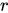is the degree of the primitive generator polynomial, then the maximal total block length is $2^{r}-1$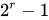, and the associated code is able to detect any single-bit or double-bit errors. We can improve this situation. If we use the generator polynomial $g(x)=p(x)(1+x)$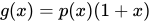, where $p$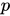is a primitive polynomial of degree $r-1$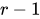, then the maximal total block length is $2^{r-1}-1$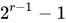, and the code is able to detect single, double, triple and any odd number of errors.

A polynomial $g(x)$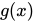that admits other factorizations may be chosen then so as to balance the maximal total blocklength with a desired error detection power. The BCH codes are a powerful class of such polynomials. They subsume the two examples above. Regardless of the reducibility properties of a generator polynomial of degree r, if it includes the "+1" term, the code will be able to detect error patterns that are confined to a window of r contiguous bits. These patterns are called "error bursts".

## Specification

The concept of the CRC as an error-detecting code gets complicated when an implementer or standards committee uses it to design a practical system. Here are some of the complications:

• Sometimes an implementation prefixes a fixed bit pattern to the bitstream to be checked. This is useful when clocking errors might insert 0-bits in front of a message, an alteration that would otherwise leave the check value unchanged.
• Usually, but not always, an implementation appends n 0-bits (n being the size of the CRC) to the bitstream to be checked before the polynomial division occurs. Such appending is explicitly demonstrated in the Computation of CRC article. This has the convenience that the remainder of the original bitstream with the check value appended is exactly zero, so the CRC can be checked simply by performing the polynomial division on the received bitstream and comparing the remainder with zero. Due to the associative and commutative properties of the exclusive-or operation, practical table driven implementations can obtain a result numerically equivalent to zero-appending without explicitly appending any zeroes, by using an equivalent, faster algorithm that combines the message bitstream with the stream being shifted out of the CRC register.
• Sometimes an implementation exclusive-ORs a fixed bit pattern into the remainder of the polynomial division.
• Bit order: Some schemes view the low-order bit of each byte as "first", which then during polynomial division means "leftmost", which is contrary to our customary understanding of "low-order". This convention makes sense when serial-port transmissions are CRC-checked in hardware, because some widespread serial-port transmission conventions transmit bytes least-significant bit first.
• Byte order: With multi-byte CRCs, there can be confusion over whether the byte transmitted first (or stored in the lowest-addressed byte of memory) is the least-significant byte (LSB) or the most-significant byte (MSB). For example, some 16-bit CRC schemes swap the bytes of the check value.
• Omission of the high-order bit of the divisor polynomial: Since the high-order bit is always 1, and since an n-bit CRC must be defined by an (n + 1)-bit divisor which overflows an n-bit register, some writers assume that it is unnecessary to mention the divisor's high-order bit.
• Omission of the low-order bit of the divisor polynomial: Since the low-order bit is always 1, authors such as Philip Koopman represent polynomials with their high-order bit intact, but without the low-order bit (the $x^{0}$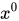or 1 term). This convention encodes the polynomial complete with its degree in one integer.

These complications mean that there are three common ways to express a polynomial as an integer: the first two, which are mirror images in binary, are the constants found in code; the third is the number found in Koopman's papers. In each case, one term is omitted. So the polynomial $x^{4}+x+1$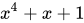may be transcribed as:

• 0x3 = 0b0011, representing $x^{4}+(0x^{3}+0x^{2}+1x^{1}+1x^{0})$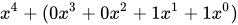(MSB-first code)
• 0xC = 0b1100, representing $(1x^{0}+1x^{1}+0x^{2}+0x^{3})+x^{4}$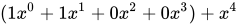(LSB-first code)
• 0x9 = 0b1001, representing $(1x^{4}+0x^{3}+0x^{2}+1x^{1})+x^{0}$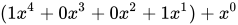(Koopman notation)

In the table below they are shown as:

Examples of CRC representations
Name Normal Reversed Reversed reciprocal
CRC-4 0x3 0xC 0x9

## Obfuscation

CRCs in proprietary protocols might be obfuscated by using a non-trivial initial value and a final XOR, but these techniques do not add cryptographic strength to the algorithm and can be reverse engineered using straightforward methods.

## Standards and common use

Numerous varieties of cyclic redundancy checks have been incorporated into technical standards. By no means does one algorithm, or one of each degree, suit every purpose; Koopman and Chakravarty recommend selecting a polynomial according to the application requirements and the expected distribution of message lengths. The number of distinct CRCs in use has confused developers, a situation which authors have sought to address. There are three polynomials reported for CRC-12, twenty-two conflicting definitions of CRC-16, and seven of CRC-32.

The polynomials commonly applied are not the most efficient ones possible. Since 1993, Koopman, Castagnoli and others have surveyed the space of polynomials between 3 and 64 bits in size, finding examples that have much better performance (in terms of Hamming distance for a given message size) than the polynomials of earlier protocols, and publishing the best of these with the aim of improving the error detection capacity of future standards. In particular, iSCSI and SCTP have adopted one of the findings of this research, the CRC-32C (Castagnoli) polynomial.

The design of the 32-bit polynomial most commonly used by standards bodies, CRC-32-IEEE, was the result of a joint effort for the Rome Laboratory and the Air Force Electronic Systems Division by Joseph Hammond, James Brown and Shyan-Shiang Liu of the Georgia Institute of Technology and Kenneth Brayer of the Mitre Corporation. The earliest known appearances of the 32-bit polynomial were in their 1975 publications: Technical Report 2956 by Brayer for Mitre, published in January and released for public dissemination through DTIC in August, and Hammond, Brown and Liu's report for the Rome Laboratory, published in May. Both reports contained contributions from the other team. During December 1975, Brayer and Hammond presented their work in a paper at the IEEE National Telecommunications Conference: the IEEE CRC-32 polynomial is the generating polynomial of a Hamming code and was selected for its error detection performance. Even so, the Castagnoli CRC-32C polynomial used in iSCSI or SCTP matches its performance on messages from 58 bits to 131 kbits, and outperforms it in several size ranges including the two most common sizes of Internet packet. The ITU-T G.hn standard also uses CRC-32C to detect errors in the payload (although it uses CRC-16-CCITT for PHY headers).

CRC-32C computation is implemented in hardware as an operation (CRC32) of SSE4.2 instruction set, first introduced in Intel processors' Nehalem microarchitecture. ARM AArch64 architecture also provides hardware acceleration for both CRC-32 and CRC-32C operations.

## Polynomial representations of cyclic redundancy checks

The table below lists only the polynomials of the various algorithms in use. Variations of a particular protocol can impose pre-inversion, post-inversion and reversed bit ordering as described above. For example, the CRC32 used in Gzip and Bzip2 use the same polynomial, but Gzip employs reversed bit ordering, while Bzip2 does not. Note that even parity polynomials in GF(2) with degree greater than 1 are never primitive. Even parity polynomial marked as primitive in this table represent a primitive polynomial multiplied by $\left(x+1\right)$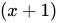. The most significant bit of a polynomial is always 1, and is not shown in the hex representations.

Name Uses Polynomial representations Parity Primitive Maximum bits of payload by Hamming distance
Normal Reversed Reciprocal Reversed reciprocal ≥ 16 15 14 13 12 11 10 9 8 7 6 5 4 3 2
CRC-1 most hardware; also known as parity bit 0x1 0x1 0x1 0x1 even
$x+1$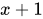CRC-3-GSM mobile networks 0x3 0x6 0x5 0x5 odd yes  4
$x^{3}+x+1$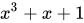CRC-4-ITU ITU-T G.704, p. 12 0x3 0xC 0x9 0x9 odd
$x^{4}+x+1$CRC-5-EPC Gen 2 RFID 0x09 0x12 0x05 0x14 odd
$x^{5}+x^{3}+1$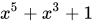CRC-5-ITU ITU-T G.704, p. 9 0x15 0x15 0x0B 0x1A even
$x^{5}+x^{4}+x^{2}+1$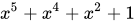CRC-5-USB USB token packets 0x05 0x14 0x09 0x12 odd
$x^{5}+x^{2}+1$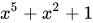CRC-6-CDMA2000-A mobile networks 0x27 0x39 0x33 0x33 odd
CRC-6-CDMA2000-B mobile networks 0x07 0x38 0x31 0x23 even
CRC-6-DARC Data Radio Channel 0x19 0x26 0x0D 0x2C even
CRC-6-GSM mobile networks 0x2F 0x3D 0x3B 0x37 even yes  1 1 25 25
$x^{6}+x^{5}+x^{3}+x^{2}+x+1$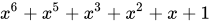CRC-6-ITU ITU-T G.704, p. 3 0x03 0x30 0x21 0x21 odd
$x^{6}+x+1$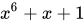CRC-7 telecom systems, ITU-T G.707, ITU-T G.832, MMC, SD 0x09 0x48 0x11 0x44 odd
$x^{7}+x^{3}+1$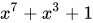CRC-7-MVB Train Communication Network, IEC 60870-5 0x65 0x53 0x27 0x72 odd
CRC-8 DVB-S2 0xD5 0xAB 0x57 0xEA even no  2 2 85 85
$x^{8}+x^{7}+x^{6}+x^{4}+x^{2}+1$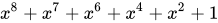CRC-8-AUTOSAR automotive integration, OpenSafety 0x2F 0xF4 0xE9 0x97 even yes  3 3 119 119
$x^{8}+x^{5}+x^{3}+x^{2}+x+1$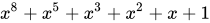CRC-8-Bluetooth wireless connectivity 0xA7 0xE5 0xCB 0xD3 even
$x^{8}+x^{7}+x^{5}+x^{2}+x+1$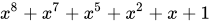CRC-8-CCITT ITU-T I.432.1 (02/99); ATM HEC, ISDN HEC and cell delineation, SMBus PEC 0x07 0xE0 0xC1 0x83 even
$x^{8}+x^{2}+x+1$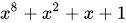CRC-8-Dallas/Maxim 1-Wire bus 0x31 0x8C 0x19 0x98 even
$x^{8}+x^{5}+x^{4}+1$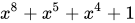CRC-8-DARC Data Radio Channel 0x39 0x9C 0x39 0x9C odd
$x^{8}+x^{5}+x^{4}+x^{3}+1$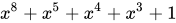CRC-8-GSM-B mobile networks 0x49 0x92 0x25 0xA4 even
$x^{8}+x^{6}+x^{3}+1$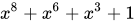CRC-8-SAE J1850 AES3; OBD 0x1D 0xB8 0x71 0x8E odd
$x^{8}+x^{4}+x^{3}+x^{2}+1$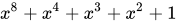CRC-8-WCDMA mobile networks 0x9B 0xD9 0xB3 0xCD even
$x^{8}+x^{7}+x^{4}+x^{3}+x+1$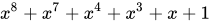CRC-10 ATM; ITU-T I.610 0x233 0x331 0x263 0x319 even
$x^{10}+x^{9}+x^{5}+x^{4}+x+1$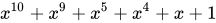CRC-10-CDMA2000 mobile networks 0x3D9 0x26F 0x0DF 0x3EC even
CRC-10-GSM mobile networks 0x175 0x2BA 0x175 0x2BA odd
CRC-11 FlexRay 0x385 0x50E 0x21D 0x5C2 even
$x^{11}+x^{9}+x^{8}+x^{7}+x^{2}+1$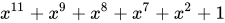CRC-12 telecom systems 0x80F 0xF01 0xE03 0xC07 even
$x^{12}+x^{11}+x^{3}+x^{2}+x+1$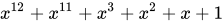CRC-12-CDMA2000 mobile networks 0xF13 0xC8F 0x91F 0xF89 even
CRC-12-GSM mobile networks 0xD31 0x8CB 0x197 0xE98 odd
CRC-13-BBC Time signal, Radio teleswitch 0x1CF5 0x15E7 0x0BCF 0x1E7A even
$x^{13}+x^{12}+x^{11}+x^{10}+x^{7}+x^{6}+x^{5}+x^{4}+x^{2}+1$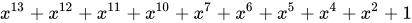CRC-14-DARC Data Radio Channel 0x0805 0x2804 0x1009 0x2402 even
CRC-14-GSM mobile networks 0x202D 0x2D01 0x1A03 0x3016 even
CRC-15-CAN 0xC599 0x4CD1 0x19A3 0x62CC even
$x^{15}+x^{14}+x^{10}+x^{8}+x^{7}+x^{4}+x^{3}+1$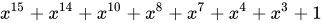CRC-15-MPT1327  0x6815 0x540B 0x2817 0x740A odd
CRC-16-Chakravarty Optimal for payloads ≤64 bits 0x2F15 0xA8F4 0x51E9 0x978A odd
CRC-16-ARINC ACARS applications 0xA02B 0xD405 0xA80B 0xD015 odd
CRC-16-CCITT X.25, V.41, HDLC FCS, XMODEM, Bluetooth, PACTOR, SD, DigRF, many others; known as CRC-CCITT 0x1021 0x8408 0x811 0x8810 even
$x^{16}+x^{12}+x^{5}+1$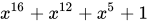CRC-16-CDMA2000 mobile networks 0xC867 0xE613 0xCC27 0xE433 odd
CRC-16-DECT cordless telephones 0x0589 0x91A0 0x2341 0x82C4 even
$x^{16}+x^{10}+x^{8}+x^{7}+x^{3}+1$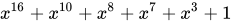CRC-16-T10-DIF SCSI DIF 0x8BB7 0xEDD1 0xDBA3 0xC5DB odd
$x^{16}+x^{15}+x^{11}+x^{9}+x^{8}+x^{7}+x^{5}+x^{4}+x^{2}+x+1$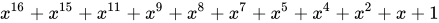CRC-16-DNP DNP, IEC 870, M-Bus 0x3D65 0xA6BC 0x4D79 0x9EB2 even
$x^{16}+x^{13}+x^{12}+x^{11}+x^{10}+x^{8}+x^{6}+x^{5}+x^{2}+1$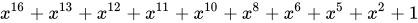CRC-16-IBM Bisync, Modbus, USB, ANSI X3.28, SIA DC-07, many others; also known as CRC-16 and CRC-16-ANSI 0x8005 0xA001 0x4003 0xC002 even
$x^{16}+x^{15}+x^{2}+1$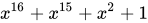CRC-16-OpenSafety-A safety fieldbus 0x5935 0xAC9A 0x5935 0xAC9A odd
CRC-16-OpenSafety-B safety fieldbus 0x755B 0xDAAE 0xB55D 0xBAAD odd
CRC-16-Profibus fieldbus networks 0x1DCF 0xF3B8 0xE771 0x8EE7 odd
Fletcher-16 Used in Adler-32 A & B Checksums Often confused to be a CRC, but actually a checksum; see Fletcher's checksum
CRC-17-CAN CAN FD 0x1685B 0x1B42D 0x1685B 0x1B42D even
CRC-21-CAN CAN FD 0x102899 0x132281 0x064503 0x18144C even
CRC-24 FlexRay 0x5D6DCB 0xD3B6BA 0xA76D75 0xAEB6E5 even
$x^{24}+x^{22}+x^{20}+x^{19}+x^{18}+x^{16}+x^{14}+x^{13}+x^{11}+x^{10}+x^{8}+x^{7}+x^{6}+x^{3}+x+1$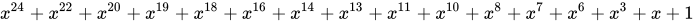CRC-24-Radix-64 OpenPGP, RTCM104v3 0x864CFB 0xDF3261 0xBE64C3 0xC3267D even
$x^{24}+x^{23}+x^{18}+x^{17}+x^{14}+x^{11}+x^{10}+x^{7}+x^{6}+x^{5}+x^{4}+x^{3}+x+1$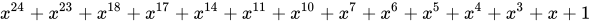CRC-24-WCDMA Used in OS-9 RTOS. Residue = 0x800FE3. 0x800063 0xC60001 0x8C0003 0xC00031 even yes 4 4 8388583 8388583
$x^{24}+x^{23}+x^{6}+x^{5}+x+1$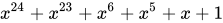CRC-30 CDMA 0x2030B9C7 0x38E74301 0x31CE8603 0x30185CE3 even
$x^{30}+x^{29}+x^{21}+x^{20}+x^{15}+x^{13}+x^{12}+x^{11}+x^{8}+x^{7}+x^{6}+x^{2}+x+1$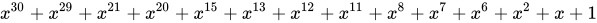CRC-32 ISO 3309 (HDLC), ANSI X3.66 (ADCCP), FIPS PUB 71, FED-STD-1003, ITU-T V.42, ISO/IEC/IEEE 802-3 (Ethernet), SATA, MPEG-2, PKZIP, Gzip, Bzip2, POSIX cksum, PNG, ZMODEM, many others 0x04C11DB7 0xEDB88320 0xDB710641 0x82608EDB odd yes 10 12 21 34 57 91 171 268 2974 91607 4294967263
$x^{32}+x^{26}+x^{23}+x^{22}+x^{16}+x^{12}+x^{11}+x^{10}+x^{8}+x^{7}+x^{5}+x^{4}+x^{2}+x+1$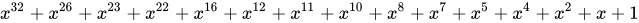CRC-32C (Castagnoli) iSCSI, SCTP, G.hn payload, SSE4.2, Btrfs, ext4, Ceph 0x1EDC6F41 0x82F63B78 0x05EC76F1 0x8F6E37A0 even yes 6 8 20 47 177 5243 2147483615
$x^{32}+x^{28}+x^{27}+x^{26}+x^{25}+x^{23}+x^{22}+x^{20}+x^{19}+x^{18}+x^{14}+x^{13}+x^{11}+x^{10}+x^{9}+x^{8}+x^{6}+1$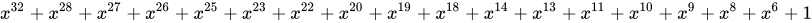CRC-32K (Koopman {1,3,28}) Excellent at Ethernet frame length, poor performance with long files 0x741B8CD7 0xEB31D82E 0xD663B05D 0xBA0DC66B even no 2 4 16 18 152 16360 114663
$x^{32}+x^{30}+x^{29}+x^{28}+x^{26}+x^{20}+x^{19}+x^{17}+x^{16}+x^{15}+x^{11}+x^{10}+x^{7}+x^{6}+x^{4}+x^{2}+x+1$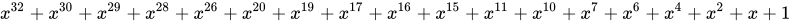CRC-32K2 (Koopman {1,1,30}) Excellent at Ethernet frame length, poor performance with long files 0x32583499 0x992C1A4C 0x32583499 0x992C1A4C even no 3 16 26 134 32738 65506
CRC-32Q aviation; AIXM 0x814141AB 0xD5828281 0xAB050503 0xC0A0A0D5 even
$x^{32}+x^{31}+x^{24}+x^{22}+x^{16}+x^{14}+x^{8}+x^{7}+x^{5}+x^{3}+x+1$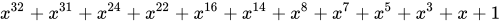Adler-32 Often confused to be a CRC, but actually a checksum; see Adler-32
CRC-40-GSM GSM control channel 0x0004820009 0x9000412000 0x2000824001 0x8002410004 even
$x^{40}+x^{26}+x^{23}+x^{17}+x^{3}+1=(x^{23}+1)(x^{17}+x^{3}+1)$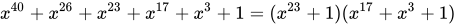CRC-64-ECMA ECMA-182 p. 51, XZ Utils 0x42F0E1EBA9EA3693 0xC96C5795D7870F42 0x92D8AF2BAF0E1E85 0xA17870F5D4F51B49 even
$x^{64}+x^{62}+x^{57}+x^{55}+x^{54}+x^{53}+x^{52}+x^{47}+x^{46}+x^{45}+x^{40}+x^{39}+x^{38}+x^{37}+x^{35}+x^{33}+$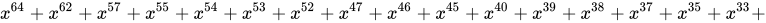$x^{32}+x^{31}+x^{29}+x^{27}+x^{24}+x^{23}+x^{22}+x^{21}+x^{19}+x^{17}+x^{13}+x^{12}+x^{10}+x^{9}+x^{7}+x^{4}+x+1$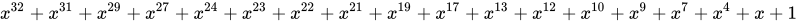CRC-64-ISO ISO 3309 (HDLC), Swiss-Prot/TrEMBL; considered weak for hashing 0x000000000000001B 0xD800000000000000 0xB000000000000001 0x800000000000000D odd
$x^{64}+x^{4}+x^{3}+x+1$